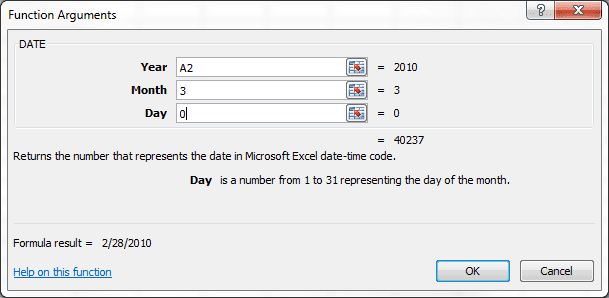# Use the DATE Function to Find the Last Day of a Month

Zero has a special meaning in the Excel date system and can be used in the DATE Function to return the last day of the month. The simplest way to show this is to use a formula to find the last day of February in the coming years.

## How to Find in Excel the Last Day of Month

If you want to find in Excel the last day of month with cell A2 containing the current year 2010, the following formula is entered into cell B2:

=DATE(A2,3,0)

and returns the result 2/28/2010.

There are three arguments for the DATE Function: Year, Month, Day. Notice that the Month argument is 3, which is March, and the Day argument is zero (0).The DATE Function will return the last day of the month prior to the Month argument, by using zero (0) for the Day argument.

### Bonus Formula – The DAY Function

To see how many days are in February — shown above in column C  — wrap the previous formula inside the DAY Function.

=DAY(DATE(A2,3,0))## 3 thoughts on “Use the DATE Function to Find the Last Day of a Month”

1.Chandoo

Wow.. I did not know that…

1.Gregory

Subtracting a day from any Date value results in the day before, and subtracting 1 from the 1st day of the month is the same as 1 -1 = 0.